## The Sunday Blog: Data manipulation

### Packing and saving space

Recently, I stumbled over a post in a Nextion related group on Facebook where the poster (let’s call him M.) asked for help. His project has a bunch of numeric settings to remember between power cycles and he ran out of EEPROM space. A discussion began and went soon in a good direction: Saving for example the state of a dual state button whose .val attribute can take the values 0 or 1 as an 32bit integer is a huge waste of space. For storing one single bit, you’d use 32 bits or 4 bytes. Now, our beloved Nextion HMIs don’t give us too much choice. Writing data to the internal EEPROM with the wepo command is possible, either for text with a variable length from 1 to 1023 bytes (not interesting in this use case), or for integers which eat up 4 bytes each (not efficient in this use case). Thus, M. was pointed towards packing multiple data entities into a single 32bit integer, using bit manipulation and shifting. That was the moment when I thought that it was time to talk about this in the next Sunday Blog…

But that was also the moment for M. to admit that he, as a hobbyist, had never learned about bit manipulation and he needed more help to understand this. So, I decided to make two parts from this. Today, we’ll look into the theory of bitwise AND, OR, XOR, NOT, and shifting right and left. And next week, we’ll build a HMI project which saves the state of around 10 dual state buttons and a few sliders together in a single 32bit integer to the EEPROM (packing) and which reads afterwards this number to restore the state of our 12+ components (unpacking).

#### Logical bit operators

There are basically 4 logical operators, AND, OR, XOR, and NOT. These can do miracles, either alone or in combination, as those people who think in CPU registers to write assembler code might already know. For all the others, here a short introduction:

Everything starts with one or two single bits which can be 0 or 1, each. The simplest operator is the NOT operator which simply returns the opposite of the single input value. When it is 1, NOT will return 0, and when it is 0, NOT will return 1. The formal writing for this is ~1 => 0 and ~0 => 1.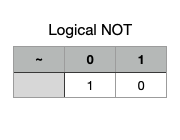Then, there is the OR operator which takes 2 operands, a, and b. It returns 1 if a is 1, or b is 1, or both. It returns only 0 if both operands are 0.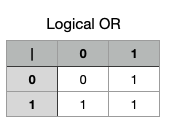The AND operator returns 1 only if a and b are 1. In all other cases, it will return 0.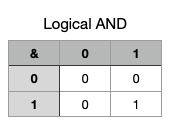Finally, there is the XOR (eXclusive OR) which returns 1 when either a or b is 1. It will return 0 if both are 1 (not exclusive), and, naturally when both are 0, too.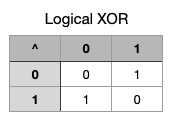#### And now, in a bigger context…

What we could do on bit level above, is also perfectly doable when you have not only one bit, but for example 8 bits in a byte or 32 bits in a word. In these cases, the operations are simply made bit for bit inside the byte or the word.

So, negating every single bit in a bigger entity consists of using the bitwise NOT operator to the whole bite or word.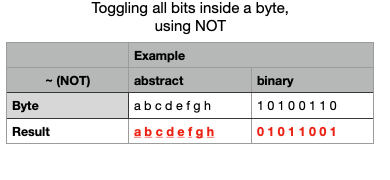This allows for example to set one or more specific bits within a byte (or word), just by bitwise OR-ing the byte (or word) with what we call a bit mask, which is simply a byte (or word) where just the corresponding bits are set to 1.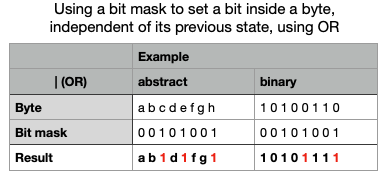In a similar way, we can use the bitwise AND operation to clear specific bits. For this, we need a bit mask where only the bits to clear are 0, and all others 1. Sometimes, it is easier to build this bit mask the other way round, only setting the corresponding bits to 1 and then flipping it around with the NOT operator. In the example below, the bit mask is 11010110 which was made by NOT-ing the bit mask from the example above, 00101001.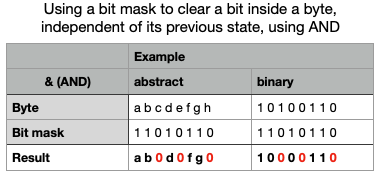The bitwise XOR allows us to toggle specific bits. Those whose corresponding bits in the bit mask are 0 remain unchanged.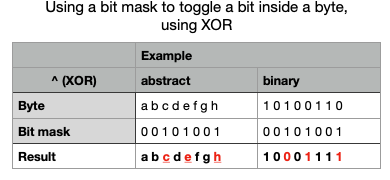#### Letting your bits dance Samba…

… or rather letting do them some sidesteps is possible by using so-called arithmetic shift operations.

Left shifting by n bit positions is noted as b = a << n. This operation shifts all bits by n steps leftwards which makes naturally that the n leftmost bits are lost, while zeroes are shifted in from the right side. In the following example, there was a shifting by 3.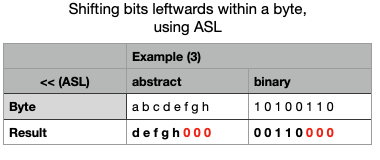Arithmetic right shifting b = a >> n is almost similar. One difference is that now, you lose the n rightmost bits. And the bits shifted in from the left are all equal to the previously leftmost bit. This is to preserve the sign of signed bytes or words. If our initial value was a signed value, its leftmost bit was the sign bit. Duplicating it n times makes sure that afterwards, the leftmost bit is still the same as before and thus, our result has the same sign.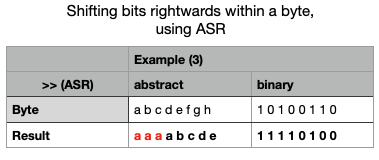#### Extracting the value of a single bit

Now, with the above operations, we have a marvelous toolbox! Let’s take a random byte with 8 bits like abcdefgh. Now, we need the value of the rightmost bit (h). Simply AND-ing it with 00000001 will give us 0000000h or simply bit h. But what if we need the fifth bit from the right (d)? We combine two operations. First, we do a right shift by 4 positions which transforms our abcdefgh into aaaaabcd, so that the bit we are interested in is again in the rightmost position. Then, again, lets AND it with 00000001 to get 0000000d or simply bit d.

#### Extracting a bit field

A bit field is nothing other then several neighbored bits within a bigger entity. Let’s say we want to extract the six bits in the middle of our example byte abcdefgh. First, we do a right shift by one to get or bit field into the rightmost position: abcdefgh >> 1 = aabcdefg. And since now, we only want the 6 rightmost bits, we AND it with a suitable bit mask: aabcdefg & 00111111 = 00bcdefg or simply bcdefg, since leading zeros can be safely ignored.

That’s it! With this knowledge, you are ready to tune in again next Sunday where we will transform this theory into practice.

Happy Nextion-ing!